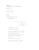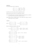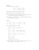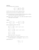# Exercise sheet 7 pdfLoading previews...matrices - eigenvalues and eigenvectorsmatrices - solving a system of linear equationsmatrices - solving a system of linear equationsmatrices - solving a system of linear equations
4 files in this resource

## Exercise sheet 7 pdf

Exercises and solutions in PDFView Item